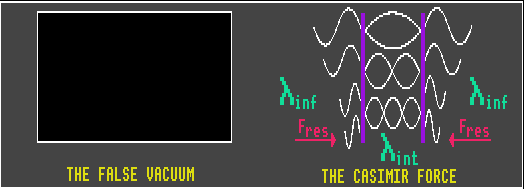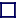### VIRTUAL PARTICLE PAIRS

Virtual particles do not satisfy Heisenbergs' Uncertainty Principle, where the energy borrowed multiplied by the time it is borrowed for must be greater than Plancks' constant, h. Particles which satisfy this relation are real particles and can be detected directly, those which do not have a fleeting existence and cannot. The heavier the virtual particle, the shorter its existence. A virtual particle of rest mass m cannot exist for longer than h/mc2, and in that time, even if it travelled at lightspeed, it can only journey a distance of h/mc, the Compton wavelength for that particle. The Compton wavelength of a proton is just 2×10-14cm, so nuclear forces are short range only. Photons have zero rest mass, so virtual photons of infinitesimally small momentum can travel infinitely large distances, and carry momentum but not energy.

Virtual photons convey the Coulomb force between two charged particles, which has infinite range (but decreases in inverse proportion to distance). Two static charges exchange virtual photons, constituting the electrostatic force between them, yet an intervening non-conductive photographic film records nothing!

Quantum Vacuum and Casimir Effect
A vacuum is not a true vacuum, but consists of a seething mass of ephemeral, continually created virtual particle/anti-particle pairs which mutually annihilate each other almost immediately. Thus the vacuum has an energy density that is non-zero, and which could be quite sizeable. Although it is impossible to observe virtual particles directly, the effects of these virtual particles can be measured as an extremely small attractive force between two parallel conductive (but chargeless) plates, where the included space excludes all wavelengths but those which are a multiple of the separation distance. The external radiation pressure on the plates is greater than the internal because of all the missing non-harmonics between the parallel plates. This is called the Casimir force. For two 1 centimeter square metal plates separated in distance by one micrometre, the Casimir force is equal to 100 nano Newtons. The same Casimir Force gives rise to the Van der Waals forces between atoms. A similar force can be measured between two parallel plates using sound waves instead of light waves.

Quantum Vacuum Fluctuations and another Big Bang explosion
The vacuum energy density is not a static value, quantum mechanics has it that it fluctuates wildly and randomly at every point in space-time. Vacuum energy has negative pressure, that is, it causes space to be pushed outwards and fuelled inflation in the early stages of the Big Bang. The expanding space would release more vacuum energy which would further accelerate the expansion of the Universe. This is called cosmic inflation. Likewise, if the energy density of the vacuum at some point in the Universe suddenly increased in value above some critically high figure, that point would start expanding exponentially, creating a new Big Bang. The probability that quantum fluctuations in the vacuum energy density will swing high enough to cause this has been calculated, and it is not zero, but luckily for us is vanishingly small, although eventually in an infinite Universe this would be a certainty.

Dark Energy and Quantum Vacuum Fluctuations
The observed energy density of the dark energy contained in the Universe has a known value. If quantum vacuum fluctuations are the same as dark energy, then the maximum frequencies of the quantum fluctuations should not exceed 1.7 THz. Experiments with the Casimir effect have shown that the quantum vacuum frequencies do exceed 1.7 THz, so that experiment excludes the possibility that dark energy and quantum vacuum fluctuations are one and the same.

Tunnelling
The quantum phenomenon of tunnelling also depends upon the creation of virtual particles. The tunnelling of microwaves through an 'impenetrable' barrier (barrier penetration) has reportedly been measured to occur at speeds above 4c, but all real photons travel at lightspeed, c, (or less).